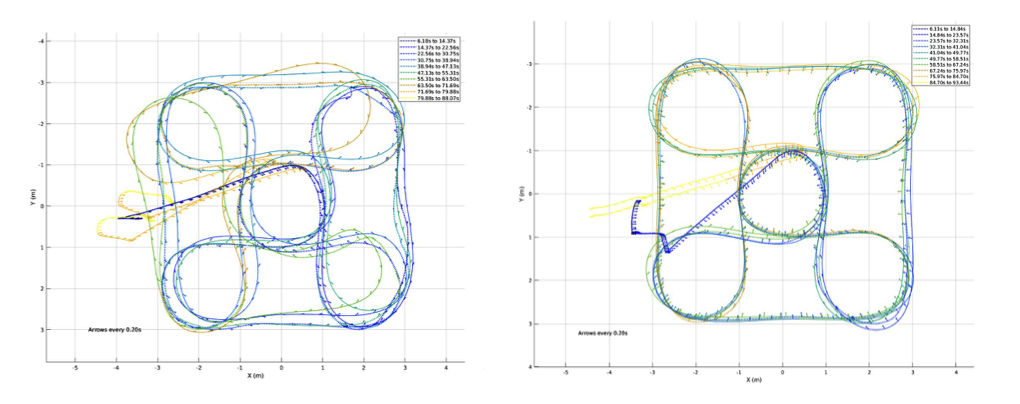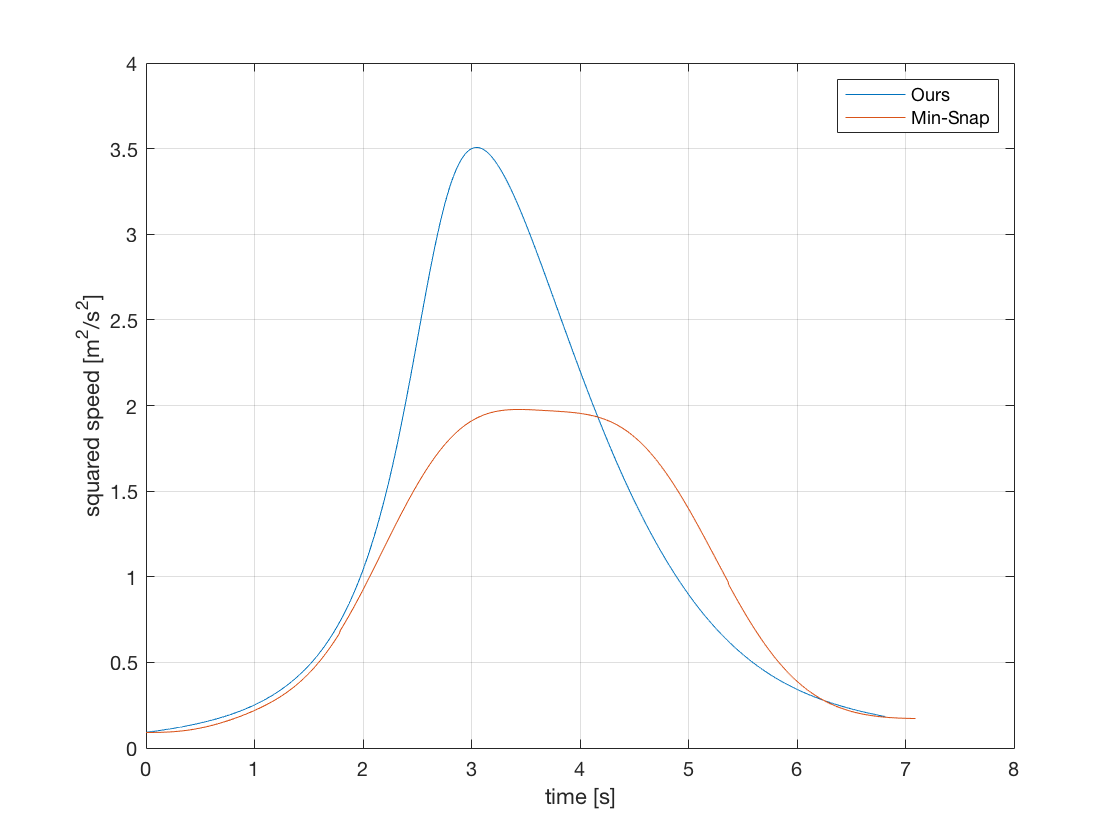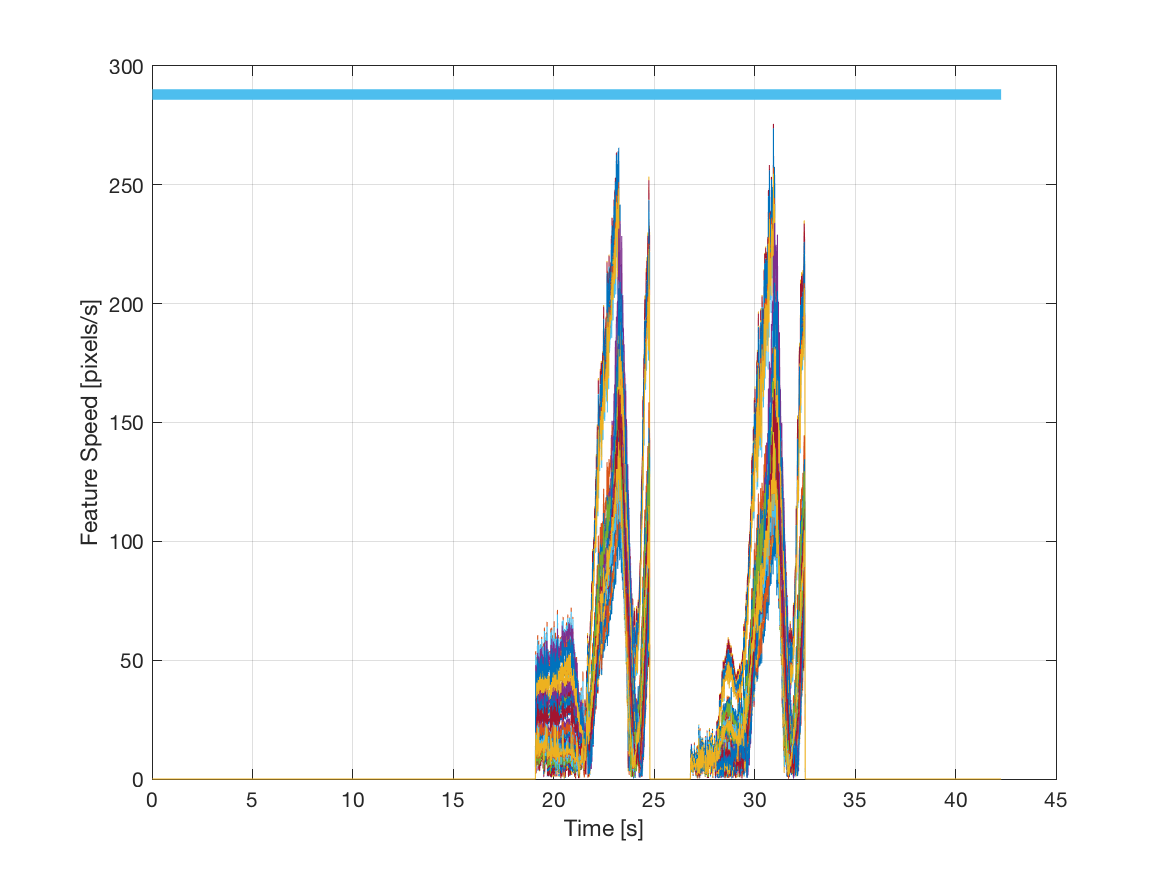### Perception-aware Motion Planning

This project studies the foundations of perception-aware planning, resulting in decision-making algorithms that optimize perception objectives. For instance, this video shows the modified yaw angle to optimize visual-inertial state estimation performance.

## Perception-aware Motion Planning

### Perception-aware Motion Planning for Quadrotor Aircraft

Most autonomous vehicles, especially high-performance aircraft, must perceive their environment very rapidly using limited computing and sensing resources. In many high-performance applications, slightly deviations in the trajectory of the vehicle may enable substantial increase in perception capabilities. In order words, the trajectory of the vehicle can be chosen carefully to optimize, not only trajectory objectives (such as, reaching the goal destination in minimum time), but also perception objectives (such as, lowering localization error). In fact, optimizing perception objectives may enable a better envelope of trajectory objectives. For example, better localization may enable the execution of even faster trajectories.

For a quadrotor aircraft, a path can be full determined by the following variables: a set of 3-degree-of-freedom waypoints, the yaw angle and the time at which we expect the aircraft to be at that point in the path. Let’s assume that the translational trajectory is provided. This is reasonable in many applications where the translation is well defined such as following a road, flying search patterns or having a preset racecourse designed by an expert. We can then tackle the other two free variables, namely yaw and speed (time allocation), to optimize perception objectives.

### Yaw Angle Selection

Assume that the speed of the aircraft is determined. Then, we are left with the problem of selecting the yaw angles along the path. If the vehicle is equipped with a forward facing camera with a limited field of view, then the selection of yaw angle is important. We developed a soft indicator function that measures the co-visibility (a measure of the duration of observing a particular landmark) that can be added to the trajectory generation problem. This then solves a local problem to generate a sequence of yaws along the trajectory that maximizes the total duration a landmark is visible along the path. Our results show that this improves the robustness of trajectory tracking for closed loop control as the desired speed is increased.

The two videos below show the yaw angle looking forward and yaw angle optimized, respectively. In the first video the vehicle flies with the vehicle looking forward along the trajectory. In the second video, the yaw angle is optimized using our approach. It is noticeable that, in the second approach the vehicle "looks" at the more feature-rich center location in the room in a way that features can be tracked (are in the camera view) for longer.

The figures below show the results of visual-inertial state estimation in both cases. The figures show the estimated trajectory using visual-inertial state estimation methods. In the first case the state estimate diverges rapidly, while the drift is much smaller in the second case.### Speed Optimization

Assume now that the yaw and translational trajectory are determined. Note that this does not determine the full pose until the time allocation is specified, since the roll and pitch are contingent on the acceleration. We show that a time parameterization determined by an efficient algorithm is indeed asymptotically optimal as the resolution of discretization is increased. The algorithm proceeds as follows. First, the upper and lower bounds of the speed parameter are estimated backwards through reachability analysis with the actuator constraints. In the forward pass, we select the maximum at the starting point and compute the maximum reachable speed in the next waypoint. This then proceeds till the speed is allocated at each waypoint. We apply this algorithm to quadrotor aircraft and show that the visibility problem is convex in this parameterization. Our experiments show that the approximations needed to apply the algorithm to quadrotor aircraft still result in valid plans.

In an experimental study, we consider a simple trajectory of circular motion to illustrate our approach. The graph below compares the speed profile returned by our algorithm and the speed profile returned by the minimization of snap.### Feature Selection

Let us consider the problem of localizing a mobile robot using visual inertial odometry. We would like to design computationally efficient algorithms to robustly estimate the ego-motion of the camera. The computational complexity of the estimation algorithm is proportional to O(n^3) where n is the number of features used in the estimation algorithm. Additionally, every feature incurs a cost to relocalize on the camera canvas from frame to frame. To reduce this load, we would like to select a fixed number of features from those available in the environment to be used in the estimation algorithm. Given a user specified trajectory, we show that a greedy algorithm is able to select k features which induce the maximal speed along the trajectory subject to continuous visibility of the feature along the trajectory and a bounded velocity of the feature on the camera canvas. We show that this approach is orders of magnitude faster and yields nearly identical results as an optimal solution from GUROBI.

The figure below shows the speed of all features in the camera image in an experiment. All features travel slower than the maximum speed allowed.